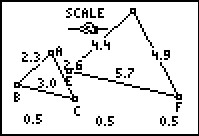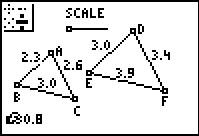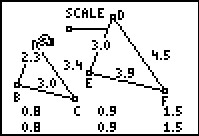••• ##### Device
• TI-83 Plus Family
• TI-84 Plus
• TI-84 Plus Silver Edition

TI Connect™

• ##### Report an Issue

Algebra I: Ratios of Similar Triangles
by Texas Instruments - Bell Ringer Lesson#### Overview

Students explore two ways of comparing side lengths of similar triangles. They will calculate ratios and change the triangles to see how the ratio changes.

#### Key Steps

•Students will explore two ways of comparing side lengths of similar triangles. First students will calculate ratios that compare corresponding side lengths.

•They can observe how the ratio changes as they move the triangle vertices and increase the shape of the similar triangle. Students will write a proportion for the three ratios.

•Then students will calculate ratios that compare two sides of the same triangle. They will write three proportions for the six ratios. The teacher should discuss how the proportions in this activity can be set up to find a missing side length.